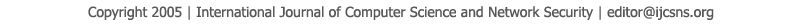To search, Click below search items.All Published Papers Search Service
 Title Author Year Keyword
 Title Determining The Pattern for 1- fault Tolerant Hamiltonian Cycle From Generalized Petersen Graph P(n,k) Author Wamiliana, F. A. M. Elfaki, Ahmad Faisol, Mustofa Usman, Isna Evi Lestari Citation Vol. 15  No. 4  pp. 26-30 Abstract Given a Generalized Petersen Graph P(n,k) a question can be asked by looking at its specific pattern whose 2n vertices and 3n edges, and its special degree on each vertices, which is 3. The degree of each vertices which is only one more than the vertices in Hamiltonian cycle makes a question: is that possible to find a relationship between Generalized Petersen Graph P(n,k) and Hamiltonian cycle, especially with 1-fault tolerant Hamiltonian where the fault occurs because either one vertex or one edge not included in the cycle. In this paper we will discuss about Generalized Petersen Graph P(n,1), if n odd, 3 ≤ n ≤ 13 and P(n,2), if n = 1(mod 6), or 3(mod 6) for 7 ≤ n ≤ 19. Keywords generalized Petersen P(n,k) graph, Hamiltonian circuit, 1-fault- tolerant Hamiltonian URL http://paper.ijcsns.org/07_book/201504/20150404.pdf